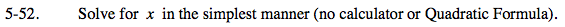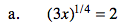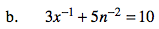### Home > PC > Chapter 5 > Lesson 5.2.1 > Problem5-52

5-52.$\left(\left ( 3x \right )^{\frac{1}{4}} \right )^{4}=2^{4}$1. Isolate the x on one side.
2. Divide by 3.
3. Get a common denominator on the right.
4. Rewrite the left side without negative exponents.
5. flip the fraction on both sides solving for x.

$3x^{-1}=10-5n^{-2}$

$x^{-1}=\frac{10}{3}-\frac{5}{3n^{2}}$

$x^{-1}=\frac{10}{3}\left ( \frac{n^{2}}{n^{2}} \right )-\frac{5}{3n^{2}}$

$x^{-1}=\frac{10n^{2}-5}{3n^{2}}$

$\frac{1}{x}=\frac{10n^{2}-5}{3n^{2}}$

$x=\frac{3n^{2}}{10n^{2}-5}$